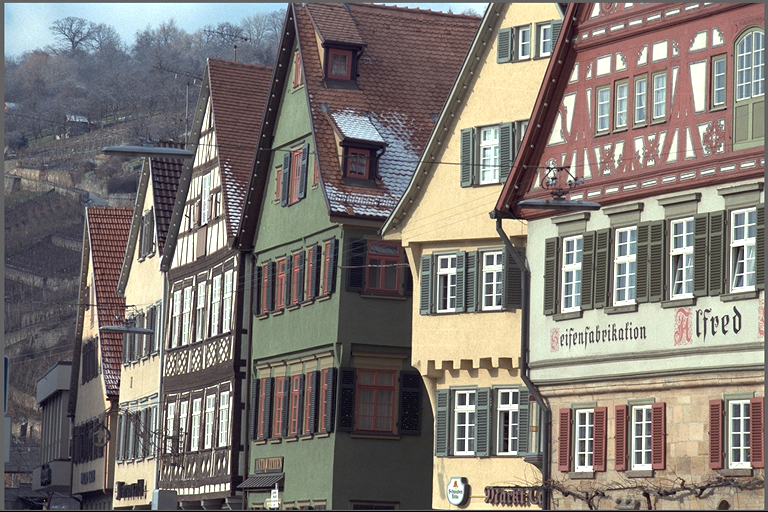Image Statistics

# Overview

The image statistics algorithm performs a two dimensional (2D) operation on the input image with the provided mask image of same dimensions. The mask defines the pixels considered for the operation. The algorithm calculates the per-channel statistics namely pixel count, sum, mean, variance, covariance of the input image masked by the mask image. Both variance and covariance are stored in a single 4x4 covariance matrix, where the diagonal is the variance and the non-diagonal elements are the covariance per-channel, considering 4 maximum number of channels.

Input Parameters OutputMask is passed as NULL (All pixels considered).
Flag is VPI_STAT_COVARIANCE

$PixelCount = 393216$

$Per-Channel Sum: \begin{bmatrix} 50316744 & 47610060 & 45611460 & 0 \\ \end{bmatrix}$

$Per-Channel Mean: \begin{bmatrix} 127.962097 & 121.078644 & 115.995941 & 0 \\ \end{bmatrix}$

$Covariance Matrix: \begin{bmatrix} 4062.606201 & 4022.210693 & 3351.239990 & 0 \\ 4022.210693 & 4263.414551 & 3651.921387 & 0\\ 3351.239990 & 3351.239990 & 3293.198730 & 0\\ 0 & 0 & 0 & 0 \end{bmatrix}$

# Implementation

The image stats works calculating various per-channel statistics of the input image defined by the mask image. The various statistics that can be calculated have their own flags. The output is stored in an array of type VPI_ARRAY_TYPE_STATISTICS. The user can choose which statistics should be calculated.

*valid - Here valid means the input image pixels that have corresponding non-zero mask pixels.

# C API functions

For list of limitations, constraints and backends that implements the algorithm, consult reference documentation of the following functions:

Function Description
vpiSubmitImageStats Returns various image statistics of the input image.

# Usage

Language:
1. Import VPI module
import vpi
2. Run Image stats algorithm on the input image using the CUDA backend.
with vpi.Backend.CUDA:
output = input.image_stats(flags=vpi.ImageStatistics.COVARIANCE)
3. Access the statistics from the output array.
stats = output.cpu().view(np.recarray)
print(stats.covariance)
1. Initialization phase
1. Include the header that defines the needed functions and structures.
Declares functions that implement image statistics algorithms.
2. Define the input image object.
VPIImage input = /*...*/;
struct VPIImageImpl * VPIImage
A handle to an image.
Definition: Types.h:256
3. Create the output array. It has to be of type VPI_ARRAY_TYPE_STATISTICS.
VPIArray output;
VPIStatus vpiArrayCreate(int32_t capacity, VPIArrayType type, uint64_t flags, VPIArray *array)
Create an empty array instance.
struct VPIArrayImpl * VPIArray
A handle to an array.
Definition: Types.h:232
@ VPI_ARRAY_TYPE_STATISTICS
VPIStats element.
Definition: ArrayType.h:83
4. Create the stream where the algorithm will be submitted for execution.
VPIStream stream;
vpiStreamCreate(0, &stream);
struct VPIStreamImpl * VPIStream
A handle to a stream.
Definition: Types.h:250
VPIStatus vpiStreamCreate(uint64_t flags, VPIStream *stream)
Create a stream instance.
5. Create the mask image to be used. It must match the dimensions of the input image.. Can be NULL if no mask is required. Has to be of format VPI_IMAGE_FORMAT_U8.
#define VPI_IMAGE_FORMAT_U8
Single plane with one 8-bit unsigned integer channel.
Definition: ImageFormat.h:100
2. Processing phase
1. Submit the algorithm for the image stats. It'll be executed by the CUDA backend.
#define VPI_STAT_COVARIANCE
Calculate full covariance matrix.
Definition: Types.h:644
VPIStatus vpiSubmitImageStats(VPIStream stream, uint64_t backend, VPIImage input, VPIArray statistics, VPIImage mask, uint32_t flags)
Returns various image statistics of the input image.
@ VPI_BACKEND_CUDA
CUDA backend.
Definition: Types.h:93
2. Optionally, wait until the processing is done.
vpiStreamSync(stream);
VPIStatus vpiStreamSync(VPIStream stream)
Blocks the calling thread until all submitted commands in this stream queue are done (queue is empty)...
3. Cleanup phase
1. Free resources held by the stream and the input and output images.
vpiArrayDestroy(output);
void vpiArrayDestroy(VPIArray array)
Destroy an array instance.
void vpiImageDestroy(VPIImage img)
Destroy an image instance.
void vpiStreamDestroy(VPIStream stream)
Destroy a stream instance and deallocate all HW resources.

For more information, see Image Statistics in the "API Reference" section of VPI - Vision Programming Interface.

# Performance

Performance benchmarks will be added at a later time.